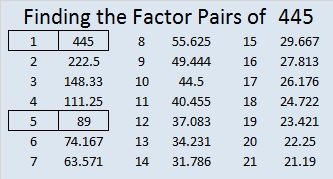# 445 and Level 4

Supposedly there is something interesting about every number. What is interesting about the number 445? When you write it in base 9, the digits are reversed. How do you convert 445 from base 10 to base 9?

Solution: The powers of 9 less than 445 in descending order are 81, 9, and 1. We first divide 445 by 81, next we divide the remainder by 9, and lastly, we divide that remainder by 1 as illustrated below.Thus 445 (base 10) = 544 (base 9).Print the puzzles or type the factors on this excel file: 10 Factors 2015-03-30

• 445 is a composite number.
• Prime factorization: 445 = 5 x 89
• The exponents in the prime factorization are 1 and 1. Adding one to each and multiplying we get (1 + 1)(1 + 1) = 2 x 2 = 4. Therefore 445 has exactly 4 factors.
• Factors of 445: 1, 5, 89, 445
• Factor pairs: 445 = 1 x 445 or 5 x 89
• 445 has no square factors that allow its square root to be simplified. √445 ≈ 21.0950445 is the hypotenuse of four Pythagorean triples:

• [267-356-445] which is [3-4-5] times 89
• [195-400-445] which is [39-80-89] times 5
• Primitive [84-437-445] (Note: 84 = 2(2 x 21) and 437 = 21^2 – 2^2, while 445 = 21^2 + 2^2)
• and Primitive [203-396-445] (Note: 396 = 2(18 x 11) and 203 = 18^2 – 11^2, while 445 = 18^2 + 11^2)